# Tangent line

(diff) ← Older revision | Latest revision (diff) | Newer revision → (diff)
Jump to: navigation, search

to a curve

A straight line representing the limiting position of the secants. Letbe a point on a curve(Fig. a). A second point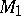is chosen onand the straight lineis drawn. The pointis regarded as fixed, andapproachesalong the curve. If, asgoes to, the line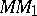tends to a limiting line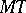, thenis called the tangent toat.Figure: t092170a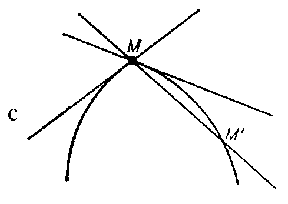Figure: t092170b

Not every continuous curve has a tangent, sinceneed not tend to a limiting position at all, or it may tend to two distinct limiting positions astends tofrom different sides of(Fig. b). If a curve in the plane with rectangular coordinates is defined by the equation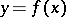andis differentiable at the point, then the slope of the tangent atis equal to the value of the derivative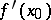at; the equation of the tangent at this point has the formThe equation of the tangent to a curve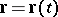in space isBy a tangent to a surfaceat a pointone means a straight line passing throughand lying in the tangent plane toat.

#### Comments

How to Cite This Entry:
Tangent line. Encyclopedia of Mathematics. URL: http://encyclopediaofmath.org/index.php?title=Tangent_line&oldid=14413
This article was adapted from an original article by BSE-3 (originator), which appeared in Encyclopedia of Mathematics - ISBN 1402006098. See original article